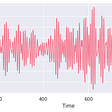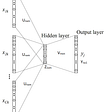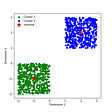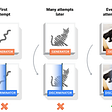# An introduction to probabilistic programming, now available in TensorFlow Probability

Posted by: Mike Shwe, Product Manager for TensorFlow Probability at Google; Josh Dillon, Software Engineer for TensorFlow Probability at Google; Bryan Seybold, Software Engineer at Google; Matthew McAteer; and Cam Davidson-Pilon.

New to probabilistic programming? New to TensorFlow Probability (TFP)? Then we’ve got something for you. Bayesian Methods for Hackers, an introductory, hands-on tutorial, is now available with examples in TFP. Available as an open-source resource for all, the TFP version complements the previous one written in PyMC3.

There’s a number of neat things about Bayesian Methods for Hackers: not only is it approachable for the probabilistic novice, but it also demonstrates how to apply probabilistic programming to real-world problems.

# Probabilistic programming for everyone

Though not required for probabilistic programming, the Bayesian approach offers an intuitive framework for representing beliefs and updating those beliefs based on new data. Bayesian Methods for Hackers teaches these techniques in a hands-on way, using TFP as a substrate. Since the book is written in Google Colab, you’re invited to run and modify the Python examples.

The TensorFlow team built TFP for data scientists, statisticians, and ML researchers and practitioners who want to encode domain knowledge to understand data and make predictions. TFP is a Python library built on TensorFlow that makes it easy to combine probabilistic models and deep learning on modern hardware. TFP allows you to:

• Evaluate different models rapidly
• Leverage modern, vectorized hardware accelerators automatically
• Launch easily and with confidence. TFP is professionally built and tested, Google-Cloud ready, and supported by a vibrant open source community.

As we discuss in related blog posts, probabilistic programming has diverse applications, including finance and the oil and gas industry. Why? Uncertainty is ubiquitous. Real-world phenomena — even those we completely understand — are subject to externalities beyond our control or even awareness. Dismissing these factors means conclusions might be wrong or at best misleading. We’ve built TFP to be accessible to all, to model the uncertainty all around us.

Many Bayesian tutorials focus on working through easy problems which have analytical solutions: think coin flips and dice rolls. While Bayesian Methods for Hackers starts with these, it quickly moves onto more realistic problems. Examples range from understanding the cosmos to detecting a change in an online user’s behavior.

In the remainder of this blog, we’ll provide an overview of a famous real-world problem that gets more detailed treatment in the Bayesian Hackers book in Chapter 2: the 1986 Space Shuttle Challenger disaster.

On January 28, 1986 on the 25th flight of the U.S. Space Shuttle, one of the two solid rocket boosters on the Shuttle Challenger exploded, due to an O-ring failure. Although mission engineers had numerous communications with the manufacturer of the O-ring about damage on previous flights, the manufacturer deemed the risk to be acceptable .

Below, we depict the observations of seven O-ring damage incidents on prior shuttle missions, as a function of ambient temperature. (At 70 degrees, there are two incidents.)

You’ll note that as the temperature decreases, there’s clearly an increase in proportion of damaged O-rings, yet there’s not an obvious temperature threshold below which the O-rings would likely fail. As with most real-world phenomena, there is uncertainty involved. We wish to determine at a given temperature t, what is the probability of an O-ring failure?

We can model the probability p of O-ring damage at temperature t using a logistic function, in particular:

where β determines the shape of the logistic function and 𝛼 is the bias term, shifting the function from left to right. Since both of these parameters can be positive or negative with no particular bounds or biases in size, we can model them as gaussian random variables:

In TFP, we can intuitively represent 𝛼 and β with `tfp.distributions.Normal`, as in this code snippet:

(To run this code snippet, head on over to the Google Colab version of Chapter 2, so you can run the entire Space Shuttle example).

Note that we get our actual value of 0 or 1 for p(t) in line 8, wherein we’re sampling from the logistic function using the previously sampled values of 𝛼 and β in lines 6 and 7. Also, note that the `evaluate()` helper function allows us to transition between graph and eager modes seamlessly, while converting tensor values to numpy. We describe eager and graph modes, as well as this helper function in more detail in the beginning of Chapter 2.

To connect the probability of failure at temperature t, p(t), to our observed data we can use a Bernoulli random variable with parameter p(t). Note that in general, Ber(p) is a random variable that takes value 1 with probability p, and 0 otherwise. So, the last part of our generative model is that we have a defect incident D𝑖 at temperature value 𝑖 that can be modeled as:

Given this generative model, we want to find model parameters so that the model can explain our observed data — that’s the goal of probabilistic inference!

TFP performs probabilistic inference by evaluating the model using an unnormalized joint log probability function. The arguments to this `joint_log_prob` are data and model state. The function returns the log of the joint probability that the parameterized model generated the observed data. To learn more about the `joint_log_prob`, please see this vignette.

For the sake of the Challenger example, here’s how we define the `joint_log_prob`:

Notice how lines 15–18 succinctly encode our generative model, one line per random variable. Also, note that `rv_alpha` and `rv_beta` represent the random variables for our prior distributions for 𝛼 and β. By contrast, `rv_observed`represents the conditional distribution for the likelihood of observations in temperature and O-ring outcome, given a logistic distribution parameterized by 𝛼 and β.

Next, we take our `joint_log_prob` function, and send it to the `tfp.mcmc` module. Markov chain Monte Carlo (MCMC) algorithms make educated guesses about the unknown input values, computing the likelihood of the set of arguments in the `joint_log_prob` function. By repeating this process many times, MCMC builds a distribution of likely parameters. Constructing this distribution is the goal of probabilistic inference.

Accordingly, we’ll set up a particular type of MCMC, called Hamiltonian Monte Carlo, over our `challenge_joint_log_prob` function:

Finally, we’ll actually perform the inference, through our `evaluate()` helper function:

Plotting the distributions for 𝛼 and β, we note that the distributions are fairly wide, as one would expect with so few data points and overlap in temperature between failure and non-failure observations. Yet, even with the wide distributions, we can be fairly confident that temperature does indeed have an effect on the probability of O-ring damage, since all of the samples of β are greater than 0. We can likewise be confident that α is significantly less than 0, since all samples are well into the negative.

As we mentioned above, what we really want to know is: What is the expected probability of O-ring damage at a given temperature? To compute this probability, we can average over all samples from the posterior to get a likely value for p(t𝑖).

We can then compute a 95% credible interval across the range of temperatures. Note that this is a credible interval, not a confidence interval typically found in frequentist approaches to statistical analysis. The 95% credible intervals tells us that we can be 95% sure that the true value will lie within the interval. For example, as we depict below with the purple region, at 50 degrees, we can be 95% sure that the probability of failure lies between 1.0 and 0.80. Ironically, many people erroneously interpret a confidence interval to have this property.

On the day of the Challenger disaster, at 31 degrees, it turns out that the posterior distribution for an O-ring failure would lead us to conclude with a high degree of confidence that a problem would occur.

This rather straightforward probabilistic analysis demonstrates the power of TFP and Bayesian methods: that they can be used to provide valuable insight and prediction into real-world problems of significant consequence.

You’ll find a cornucopia of real-world examples in the Bayesian Hackers book. An analysis of text-messaging volume over time that you can apply to a wide variety of fault detection problems in manufacturing and production systems. Software engineers at Google applied the methods for the text-messaging analysis to understanding text flakiness for production software — within a few weeks after we first drafted the TFP version of the chapter.

You’ll also find an analysis to find dark matter in the universe. Also, predicting returns of shares in public companies, i.e., stock returns.

We hope that you’ll dig into the conceptual walkthroughs in the book, applying the techniques to problems in your field. Please help us to keep this book in top shape by making comments and pull requests in the github!

# Acknowledgements

We thank the TensorFlow Probability team for their assistance in writing the TFP version of the Bayesian Hackers book.

# References

--

--

--

## More from TensorFlow

TensorFlow is an end-to-end open source platform for machine learning.

## A Glimpse into my GSoC project## Using T-SNE to Visualise how your Deep Model thinks## Build your first Random Forest classifier## Popular pretraining methods in the modern NLP world## Reinforcement Learning for Beginners: Q-Learning and SARSA## The Complete Machine Learning Lifecycle in one Platform — PrimeHub on Open-Source Spotlight## How Does DALL·E 2 Work?## K-Means Clustering Algorithm## TensorFlow

TensorFlow is a fast, flexible, and scalable open-source machine learning library for research and production.

## Kernel Density Estimation for Anomaly Detection in Python: Part 1